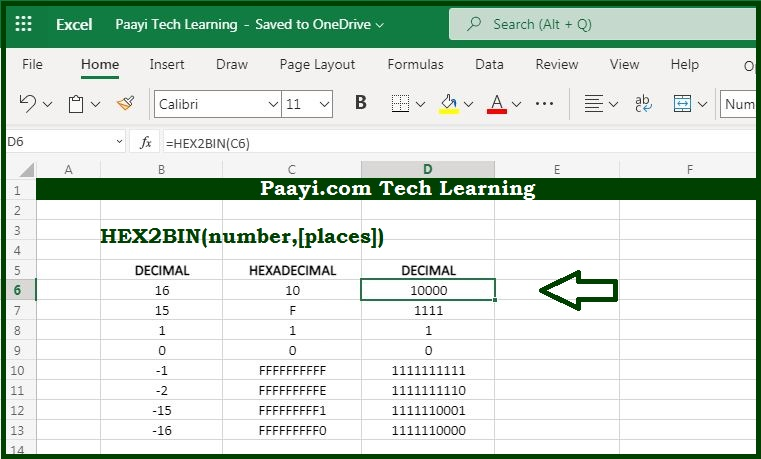# Learn How to Use Microsoft Excel HEX2BIN Function

Written by | 0 Comments | 656 Views

In this article, you will learn how to use the Microsoft Excel HEX2BIN function and its prime function in Microsoft Excel. You will also get to know the Microsoft Excel HEX2BIN function return value and syntax with the help of some examples.

Microsoft Excel HEX2BIN Function

The prime function of the Microsoft Excel HEX2BIN function is to convert hexadecimal numbers to binary numbers. That implies with the help of the HEX2BIN function you can able to convert the hexadecimal number to its binary equivalent.

Return Value of HEX2BIN Function

The return value will be the binary number.

Syntax of HEX2BIN Function

=HEX2BIN(number, [places])

Where the arguments:

number: It is the hexadecimal number that you want to convert into binary.

places: Joins the resulting binary number with zeros up to the specified number of digits. In case omitted returns the least number of characters required to represent the number (optional).

## How to Use Microsoft Excel HEX2BIN Function?As we know that HEX2BIN function used to convert hexadecimal numbers to their binary equivalent. Always keep in mind that:

Microsoft Excel only converts to binary numbers of 10 digits or less, so the input range is restricted to -512 to 511 decimal value.

The input number should be less than or equal to the ten alphanumeric characters, or the function will return the error as #NUM!.

The internal (binary) representation of the hexadecimal number uses two's complement notation. The first bit shows whether the number is positive or negative, and the rest 39 bits denote the magnitude of the number.